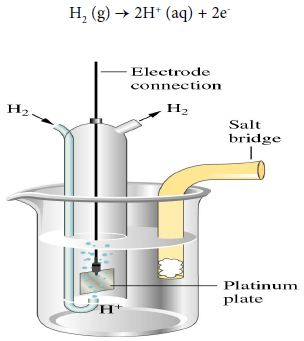# Electro-chemical Cell Notation

It is easier to have a shorthand way of writing out particular electrochemical cells. A cell consisting of the zinc-zinc ion half-cell and the copper-copper ion half-cell is written:

Zn (s) │ Zn2+ (aq) ││ Cu2+ (aq) │ Cu(s)

The anode (oxidation half-cell) is written on the left. The cathode (reduction half-cell) is written on the right.

A single vertical bar indicates a phase boundary, such as between a solid terminal and the electrolyte solution. The two electrolytes are connected by a salt bridge, denoted by two vertical bars. The cell terminals are at the extreme ends in the cell notation.

Hydrogen electrode

When the half-cell reaction involves a gas, an inert material such as platinum serves as a terminal and an electrode surface on which the reaction occurs. In a hydrogen electrode, hydrogen bubbles over a platinum plate immersed in an acidic solution.

The anode half-reaction is:

H2 (g) → 2H+ (aq) + 2eThe notation for the hydrogen electrode, written as an anode, is shown below:

Pt │ H2 (g) │ H+ (aq)││

To write such an electrode as a cathode, the notation is simply reversed as shown below:

││H+ (aq) │ H2 (g) │Pt